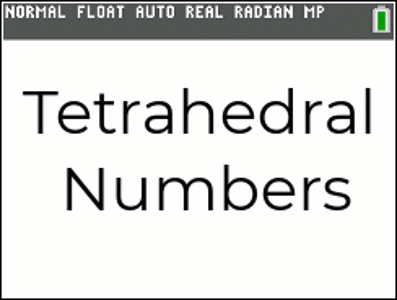# Activities

•• ##### AuthorAust Senior

50 Minutes

• ##### Device
•TI-84 Plus CE

## Part 2 of 3 - Tetrahedral Induction#### Activity Overview

Part 2 of 3: Induction for tetrahedral numbers requires students to establish a formula for the tetrahedral numbers. Just like the first activity in this series, students use a variety of techniques, but this time with much less mathematical and technology scaffolding. Students are then required to prove, by Mathematical Induction that their formula holds true. Other similar formulas are also proved using the process of Mathematical Induction.

#### Objectives

Part 2 of 3: Induction - students build equations visually, graphically and numerically and then prove their formula to be true for all whole numbers by Mathematical Induction. Additional questions are provided for students to practice the process of Mathematical Induction.

#### Vocabulary

• Induction
• Tetrahedral Numbers
• Sum
• Cubic